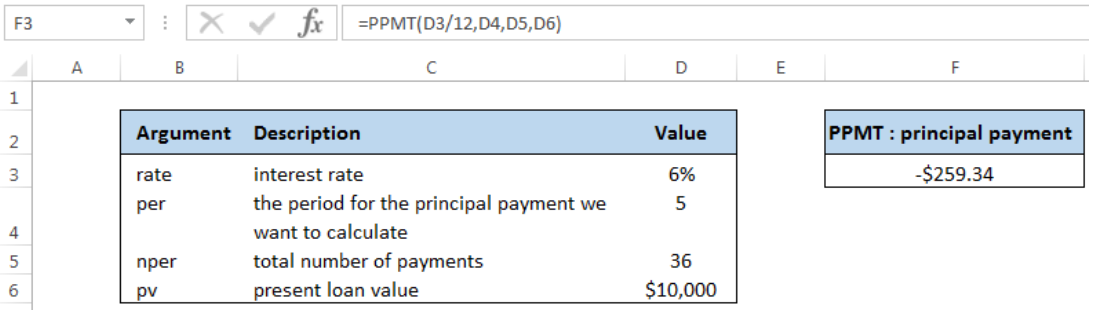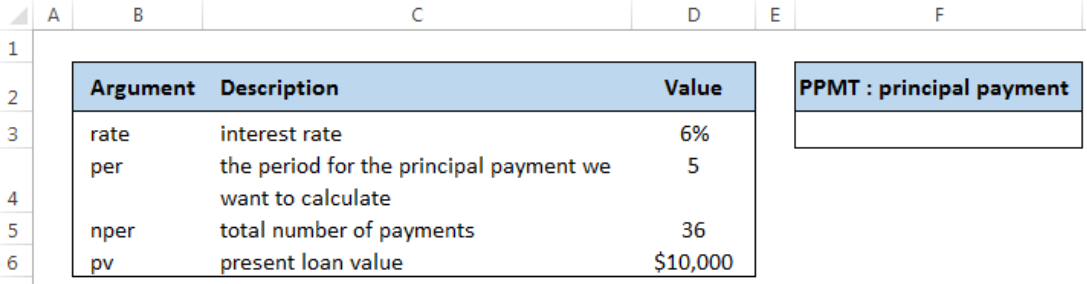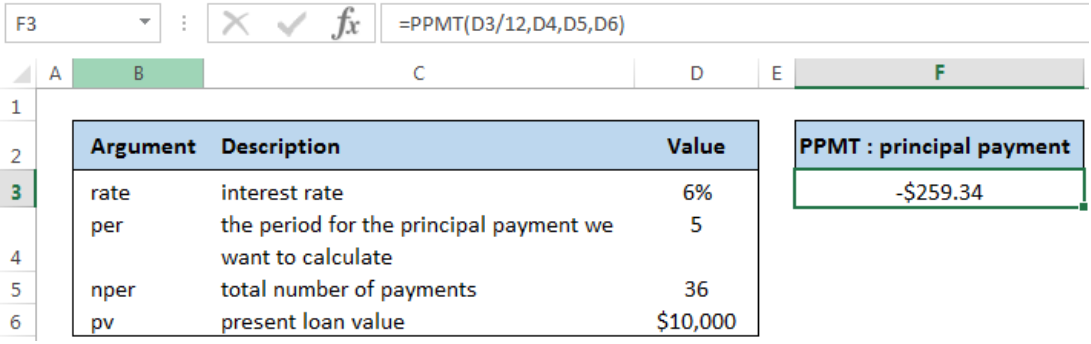Get instant live expert help with Excel or Google Sheets“My Excelchat expert helped me in less than 20 minutes, saving me what would have been 5 hours of work!”

#### Post your problem and you’ll get Expert help in seconds.

Your message must be at least 40 characters
Our professional Expert are available now. Your privacy is guaranteed.

# Calculate principal for given period

Excel allows us to calculate the principal payment for any loan using the PPMT function. This tutorial will assist all Excel users in calculating a principal portion in a loan for a given month.Figure 1. Result of PPMT function for principal payment on the 5th month

## Syntax of the PPMT Function

`=PPMT (rate, per, nper, pv, [fv], [type])`

where

• rate – the interest rate for a period of payment; if payment is monthly, rate should be annual rate divided by 12
• per – payment period for the principal payment we want to calculate
• npertotal number of payment periods
• pv – the present value of a loan
• [fv]optional; the future value of a loan after the last payment; if omitted, the default value is 0
• [type] –   optional; specifies the timing of the payment
• 0 (zero) – payment at the end of the period; also the default value
• 1 – payment at the start of the period

## Setting up the Data

Our table contains the arguments of the PPMT function, with corresponding descriptions and values.  We assign cell F3 to contain the PPMT formula.Figure 2. Sample data for the PPMT formula

## Calculate PPMT for the 5th month

We want to get the principal payment for the 5th period of payment in cell F3.

The interest rate is 6%/12, the period is 5, the total number of periods is 36 and the present loan value is \$10,000.Figure 3. Entering the PPMT formula to calculate the principal payment

Follow these steps to calculate the principal payment on the 5th month:

Step 1. Select cell F3

Step 2. Enter the formula: `=``PPMT(D3/12,D4,D5,D6)`

Step 3. Press Enter

Note :  The argument for rate is D3/12 because we must input the monthly interest rate

The formula returns the value  -\$259.34 in cell F3. This is the principal payment on the 5th month.  It is a negative value because it is an outgoing cash flow, or payment.

Most of the time, the problem you will need to solve will be more complex than a simple application of a formula or function. If you want to save hours of research and frustration, try our live Excelchat service! Our Excel Experts are available 24/7 to answer any Excel question you may have. We guarantee a connection within 30 seconds and a customized solution within 20 minutes.

### Did this post not answer your question? Get a solution from connecting with the expert.Another blog reader asked this question today on Excelchat:## Subscribe to Excelchat.coAnother blog reader asked this question today on Excelchat: